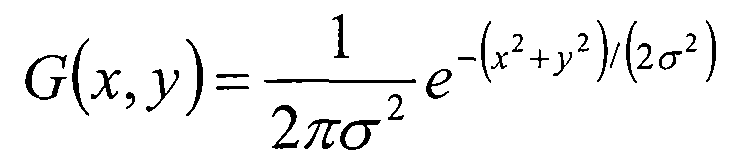image-processing
matlab80``````I=imread(image);
h=fspecial('gaussian',si,sigma);
I=im2double(I);
I=imfilter(I,h,'conv');
figure,imagesc(I),impixelinfo,title('Original Image after Convolving with gaussian'),colormap('gray');
``````

Stack Overflow收藏评论# 创建高斯蒙版``````%// Generate horizontal and vertical co-ordinates, where
%// the origin is in the middle
ind = -floor(N/2) : floor(N/2);
[X Y] = meshgrid(ind, ind);

h = exp(-(X.^2 + Y.^2) / (2*sigma*sigma));

%// Normalize so that total area (sum of all weights) is 1
h = h / sum(h(:));
``````

# 过滤图像

``````N = 5; %// Define size of Gaussian mask
sigma = 2; %// Define sigma here

ind = -floor(N/2) : floor(N/2);
[X Y] = meshgrid(ind, ind);
h = exp(-(X.^2 + Y.^2) / (2*sigma*sigma));
h = h / sum(h(:));

%// Convert filter into a column vector
h = h(:);

%// Filter our image
I = im2double(I);
C = im2col(I_pad, [N N], 'sliding');
C_filter = sum(bsxfun(@times, C, h), 1);
out = col2im(C_filter, [N N], size(I_pad), 'sliding');
``````

`out`包含对输入图像`I`应用高斯滤波蒙版后的滤波图像。例如，假设`N = 9, sigma = 4` 。我们还要使用`cameraman.tif` ，它是MATLAB系统路径的一部分。通过使用以上参数以及图像，这就是我们得到的输入和输出图像：收藏评论

•社区规范
•提出问题
•进行投票
•个人资料
•优化问题
•回答问题关于我们常见问题内容许可联系我们